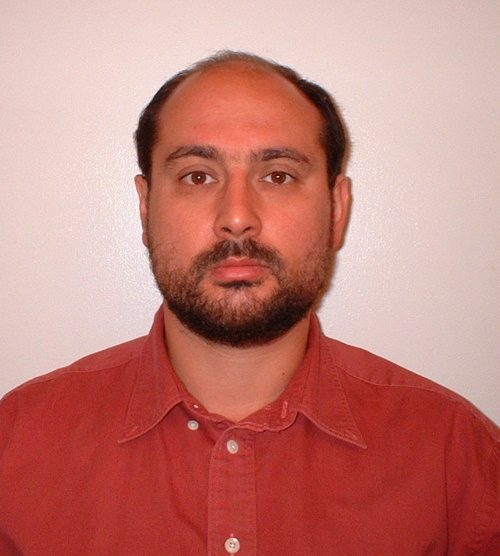I am a Professor at the Department of Mathematics and Statistics  of the University of Vermont.

Currect projects:

• Eficient iteration methods for Hartree-Fock (multi-plarticle) equations.
• Multi-channel all-optical processing and regeneration of amplitude- and phase-modulated optical signals. This project was done in collaboration with the experimental group of Prof. Michael Vasilyev from the University of Texas at Arlington.
Past projects:
• Development of higher-order schemes for the Method of characteristics for coupled-mode equations.
• Stability of numerical methods for nonlinear PDEs beyond the von Neumann analysis and "principle of frozen coefficients" (specifically, for the Split-step method and Method of characteristics).
• Numerical modeling of electromagnetic wave scattering by small particles randomly distributed in a volume, as well as by rough surfaces.  The specific application has been lighting by LEDs.
• Iteration methods for finding stationary solitary waves of nonlinear evolution equations.
• Pulse propagation in optical fibers (nonlinearity, noise accumulation, etc).
• Perturbation theory for nonlinear wave equations.
• The Inverse Scattering Transform method for soliton equations.